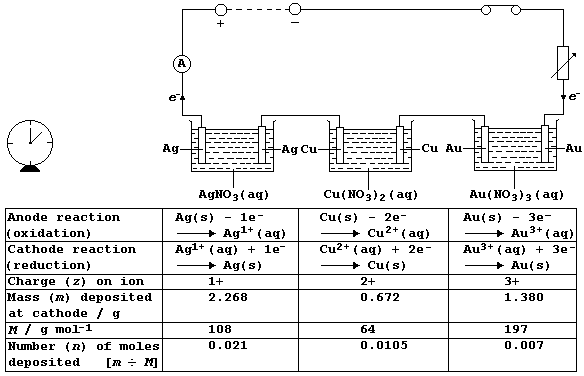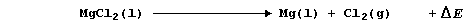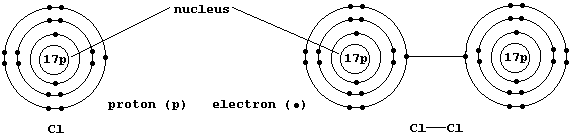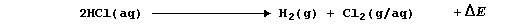```SELECTED PRINCIPLES: ELECTROLYSIS (2)
Practical applications of electrolysis include the extraction of metals
(e.g., sodium), the manufacture of compounds (e.g., sodium hydroxide),
and both the electroplating and purification of metals (e.g., copper).
These applications make use, indirectly, of two laws of electrolysis
determined by Michael Faraday (1791 - 1867). His First Law states 'that
the mass of a substance produced at an electrode during electrolysis is
proportional to the quantity of electricity passed'. And his Second Law
states 'that the quantity of electricity required to produce one mole
of a substance from its ions is proportional to the charge on those
ions'. Together, these two laws are summarized by two nifty equations,
Q = I × t  and  Q = n × z × F
where: Q, measured in coulombs (C), is the quantity of electricity;
I, measured in amps (A), is the current; t, measured in seconds (s),
is the time; n is the number of moles of substance produced at the
electrode; z is the charge on the ion; and, F is a constant, with a
value of 96500 C mol-ą.
This diagram shows a circuit, which includes three electrolytic cells
connected in series, through which a current of 0.75 A was passed for
45 minutes; so, the quantity of electricity (Q) which passed through
this circuit was:  Q = I × t = 0.75 × 45 × 60 = 2025 C. And the results
of this experiment are summarized in the Table below; its careful study
should prove rewarding.``````[Note that each anode in this experiment is an 'active' electrode; so,
overall, there is a net transfer of metal atoms from anode to cathode.]```
 ```An(ode) ... There once was a damsel called Goldilocks, whose hobby was counting odd sox. She sat in her box, and said pox on you fox, because she always got flummoxed by red and by ox. So along came three bears, who rushed up the stairs, to sort out this mess for a lass in distress. And out of the blue came some bees, if you please, to see if they could help her too. There's no need to fuss, if you want the A plus, because an ode is so easy to suss. Simply feign you're in Spain, we know it's insane, and sing in the rain ... Olé! Honey!```

```1.  The subject of the equation     Q = n × z × F     is the quantity
of electricity (Q). Rearrange this equation so that its subject is the
number of moles (n) of substance produced. ____________________________
The subject of the equation     Q = I × t     is also Q. Rearrange this
equation so that its subject is the current (I). ______________________

2.  Sea water is a rich source of magnesium ions (their concentration
is about 1.33 g dm-ł), and magnesium chloride is obtained from this
resource by concentration followed by fractional crystallization. One
method of extracting magnesium metal is by the electrolysis of molten
magnesium chloride at about 750°C; i.e.,``````A typical electrolytic cell used in industry operates at a current (I)
of 200 kA. Determine the mass (m) of magnesium metal that would be
obtained in 12 hours - as follows.
Calculate the quantity of electricity used in 12 hours. _______________
_______________________________________________________________________
Calculate the number of moles of magnesium produced at the cathode. ___
_______________________________________________________________________
And finally, calculate the mass of magnesium obtained. ________________
_______________________________________________________________________

State the number of moles of dichlorine that would be evolved at the
anode during the same 12 hour period. _________________________________

3.  Shown below are electron-structure diagrams for atomic chlorine and
molecular chlorine (or dichlorine).``````State briefly why a chlorine atom is considered to be a free-radical.
_______________________________________________________________________

4.  In an experiment executed at room temperature and pressure, using
a cell equipped with carbon-graphite electrodes and gas burettes, an
aqueous solution of hydrochloric acid was electrolyzed; i.e.,``````A current (I) of 0.75 A was passed through this electrolytic cell for a
period of time; 25.2 cmł of dihydrogen was collected over the cathode
and 23.7 cmł of dichlorine was collected over the anode. Recalling that
the volume of one mole of any gas at r.t.p. is 24000 cmł, determine the
time (t) taken to obtain this volume of dihydrogen - as follows.
Calculate the number of moles of molecular hydrogen evolved at the
cathode. ______________________________________________________________
State the number of moles of atomic hydrogen, H(g), produced at the
cathode _______________________________________________________________
Calculate the quantity of electricity required to evolve this number of
moles of hydrogen atoms. ______________________________________________
And finally, calculate the time taken for this quantity of electricity
to be used. ___________________________________________________________

Suggest one reason why the volume of gaseous dichlorine collected was
significantly less than that of dihydrogen. ___________________________
_______________________________________________________________________

Dr. R. Peters                   Next                     Contents' List```